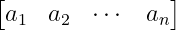# How to denote row matrix in LaTeX?

Elements of the row matrix are located along a row according to the sequence. Latex has more than one package to define the matrix. In this tutorial, row matrix is ​​define using amsmath and physics packages.

In this case, a row must be created in the latex environment. And according to the sequence, the elements of the matrix have to be inserted in this latex environment.

... & ... & ... & ...

You notice syntax above. A row is taken here and four elements are inserted in it. & symbol is used to separate each element of the row.

## amsmath package for row matrix

To be bound by brackets on both sides of the matrix, bmatrix must be passed as an argument in a latex environment.

\documentclass{article}
\usepackage{amsmath}
\begin{document}
$\begin{bmatrix} a_{1} & a_{2} & \cdots & a_{n} \end{bmatrix}$
\end{document}

Output :## physics package for row matrix

There are more than one process for defining a matrix in a physics package. However, the easiest process is to define matrix using \mqty command.

\documentclass{article}
\usepackage{physics}
\begin{document}
$\mqty[a_{1} & a_{2} & \cdots & a_{n}]$
\end{document}

Output :#### Md Jidan Mondal

LaTeX expert with over 10 years of experience in document preparation and typesetting. Specializes in creating professional documents, reports, and presentations using LaTeX.